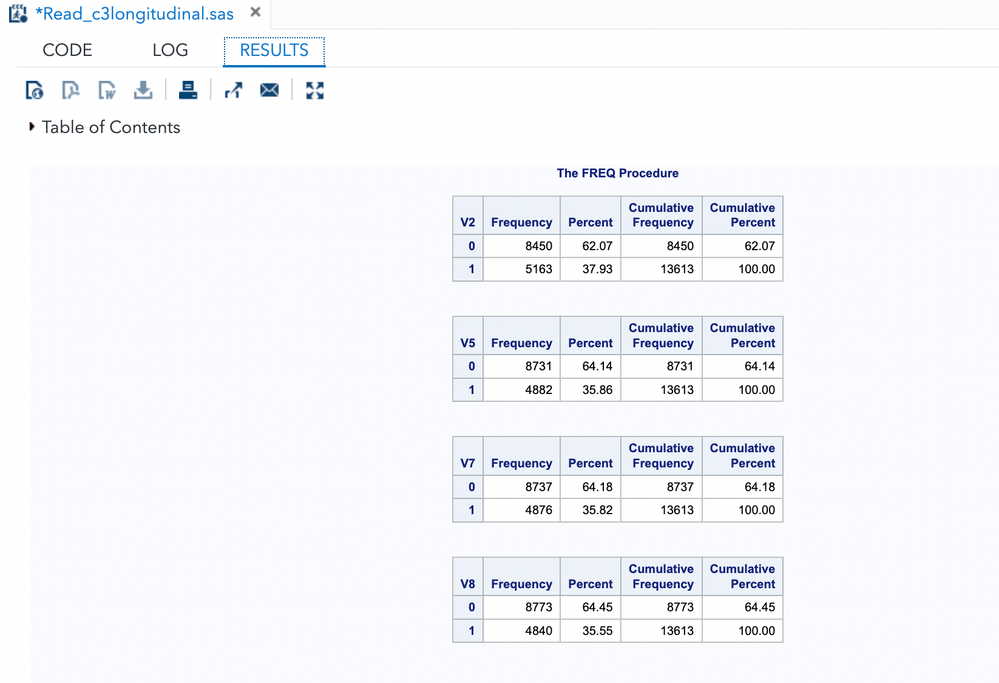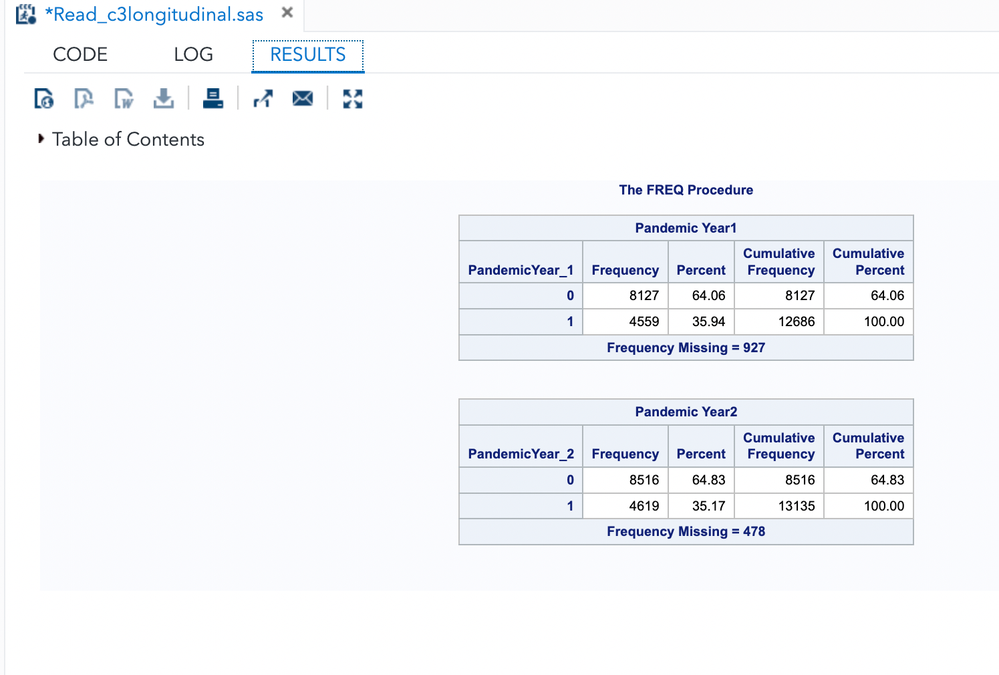## How to create new variable by combining existing two variables from same data?

Hi,

I wanted to create a new variable by combining existing two variables from the same data. I used this code. I can run it, but I am getting incorrect results. After combining, it is supposed to increase the frequency of the variable but the output results I get have lesser frequency than original two variables. Appreciate it if anyone could help with this. Thank you.

`data project_1;     set project;         *pandemic year*;     if v2 = 1 and v5 = 1 then PandemicYear_1 = 1; *pandemic year 1;      if v2 = 0 and v5 = 0 then PandemicYear_1 = 0; *none;       if v2 = .  or v5 = . then PandemicYear_1 = .;     label PandemicYear_1 = Pandemic Year1;          if v7 = 1 and v8 = 1 then PandemicYear_2 = 1; *pandemic year 2;      if v7 = 0 and v8 = 0 then PandemicYear_2 = 0; *none;       if v7 = .  or v8 = . then PandemicYear_1 = .;     label PandemicYear_2 = Pandemic Year2; run; `

8 REPLIES 8

## Re: How to create new variable by combining existing two variables from same data?

You sure those first conditions should be AND rather than an OR?

if v2=1 or v5=1???

## Re: How to create new variable by combining existing two variables from same data?

I also tired using OR. Same problem, I am able to run it but gets incorrect results.

## Re: How to create new variable by combining existing two variables from same data?

• ``````data project_1;
set project;

*pandemic year*;
if v2 = 1 or v5 = 1 then PandemicYear_1 = 1; *pandemic year 1;
else if v2 = 0 or v5 = 0 then PandemicYear_1 = 0; *none;
else if v2 = .  or v5 = . then PandemicYear_1 = .;
label PandemicYear_1 = "Pandemic Year1";

if v7 = 1 or v8 = 1 then PandemicYear_2 = 1; *pandemic year 2;
else if v7 = 0 or v8 = 0 then PandemicYear_2 = 0; *none;
else if v7 = .  or v8 = . then PandemicYear_2 = .;
label PandemicYear_2 = "Pandemic Year2";

run; ``````
• Use IF/ELSE not just IF otherwise you overwrite results.
• Labels require quotes, errors should have been generated for that statement
• In the last series there is PANDEMICYear_1 instead of 2

Try with those issues fixed and I would guess it does need to be OR

## Re: How to create new variable by combining existing two variables from same data?

I tried all of these, I still got the same result.
If we combine the two variables, the new variable supposes to have the total frequency of both variables. I still get a frequency lesser than the actual individual frequency.## Re: How to create new variable by combining existing two variables from same data?

How do you measure the frequency?  We don't see that in your program.  Remember, PROC FREQ will automatically remove observations where one of your variables is missing.

Do you really need a new variable, or do you just need to know more about the original variables?  Here's a program that will help you learn about the original variables without creating a new variable:

``````proc freq data=project;
tables v2 * v5 / missing list;
tables v7 * v8 / missing list;
run;``````

See whether that does what you need, or whether you really need to create variables.

## Re: How to create new variable by combining existing two variables from same data?

For my study, I have three outcome variables, I wanted to make it as two outcome variables. Out of three outcome variables, I am combining two of outcome variables into one variable.
I got your point now, some observations/values get deleted when we combine the variables.

Thank you for being so helpful.

## Re: How to create new variable by combining existing two variables from same data?

There are 4 ways to combine two binary variables, not 3.

``````data test;
do v1=0,1;
do v2=0,1;
v3= 2*v1 + v2;
output;
end;
end;
run;``````
```Obs    v1    v2    v3

1      0     0     0
2      0     1     1
3      1     0     2
4      1     1     3
```

If you start treating missing values as a special case then there are 3*3= 9 ways instead of just 4.

## Re: How to create new variable by combining existing two variables from same data?

Hi @Dawa93

It would be helpful if you provided a "have" and "want" dataset (see other posts for examples

Below I create a sample dataset (project) containing all possible combinations of v2, v5, v7 & v8 based on your IF logic. Then I included your sample code that creates the project_1 dataset. Finally, I've added my suggested code that creates project_2 dataset.

Now given you have observations for each of the possible values of the input variables (assuming they can only have values of 0,1 and missing (.) you can easily review the output datasets (project_1 and project_2) against the logic and figure out what you need to adjust to get the results you are looking for.

``````/* Create sample data covering all possible values */

data project ;
do v2=.,0,1 ;
do v5=.,0,1 ;
do v7=.,0,1 ;
do v8=.,0,1 ;
output ;
end ;
end ;
end;
end ;
run ;

data project_1;
set project;

*pandemic year*;
if v2 = 1 and v5 = 1 then PandemicYear_1 = 1; *pandemic year 1;
if v2 = 0 and v5 = 0 then PandemicYear_1 = 0; *none;
if v2 = .  or v5 = . then PandemicYear_1 = .;
label PandemicYear_1 = Pandemic Year1;

if v7 = 1 and v8 = 1 then PandemicYear_2 = 1; *pandemic year 2;
if v7 = 0 and v8 = 0 then PandemicYear_2 = 0; *none;
if v7 = .  or v8 = . then PandemicYear_1 = .;
label PandemicYear_2 = Pandemic Year2;
run;

/* Suggested code */
data project_2 ;
/* You only need a single label statement */
label
PandemicYear_1 = Pandemic Year1
PandemicYear_2 = Pandemic Year2;

set project ;
if v2 = 1 and v5 = 1 then PandemicYear_1 = 1; *pandemic year 1;
else if v2 = 0 and v5 = 0 then PandemicYear_1 = 0; *none;
else if v2 = .  or v5 = . then PandemicYear_1 = .;
/* need to catch potential values of v2 & v5 that are not covered above e.g. v2=1 and v5=0 */
else PandemicYear_1 = -999 ;

if v7 = 1 and v8 = 1 then PandemicYear_2 = 1; *pandemic year 2;
else if v7 = 0 and v8 = 0 then PandemicYear_2 = 0; *none;
else if v7 = .  or v8 = . then PandemicYear_1 = .; /* Should this be PandemicYear_2 */
/* need to catch potential values of v7 & v8 that are not covered above e.g. v7=1 and v8=0 */
else PandemicYear_2 = -999 ;
run; ``````
From SAS Users blog